# Solving Linear Equations Foldable Mrs Math Solve Equations

• Slides: 17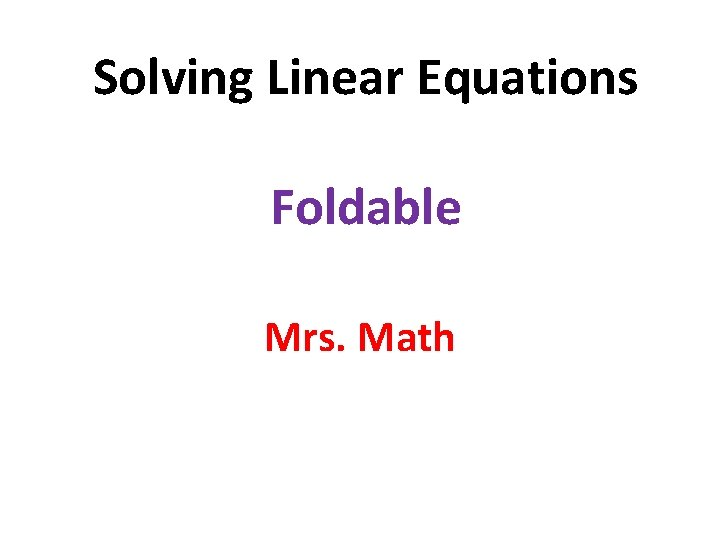Solving Linear Equations Foldable Mrs. MathSolve Equations Foldable Fold along the middle (hotdog style)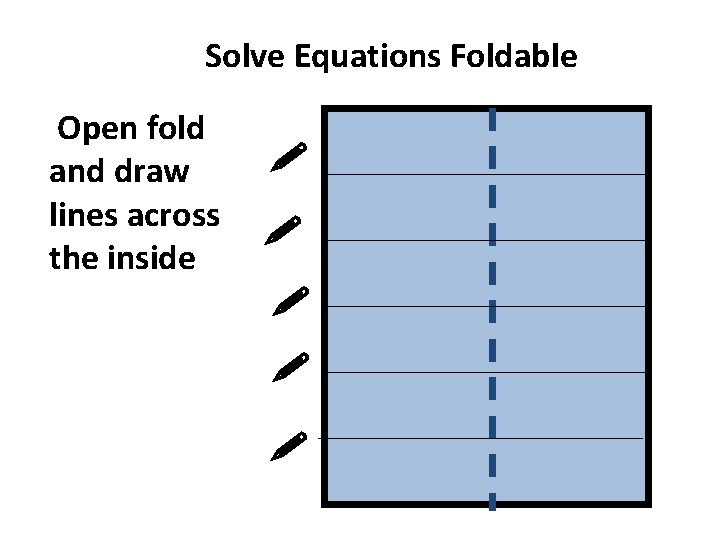Solve Equations Foldable Open fold and draw lines across the insideSolve Equations Foldable Cut to the middle fold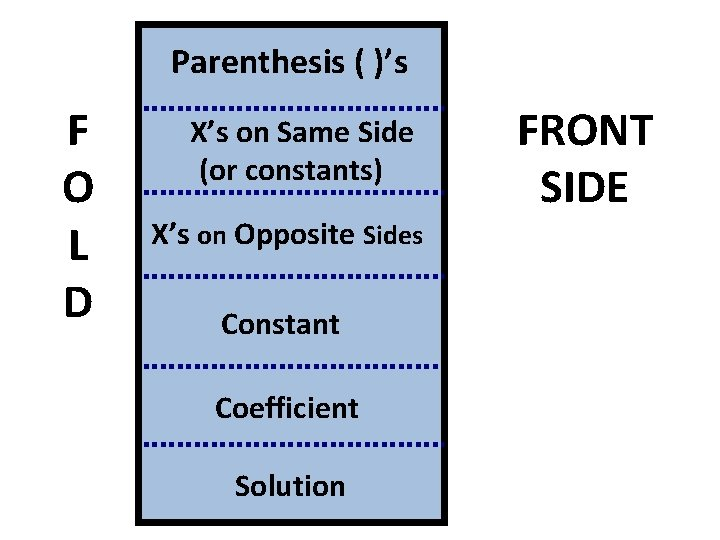Parenthesis ( )’s F O L D X’s on Same Side (or constants) X’s on Opposite Sides Constant Coefficient Solution FRONT SIDE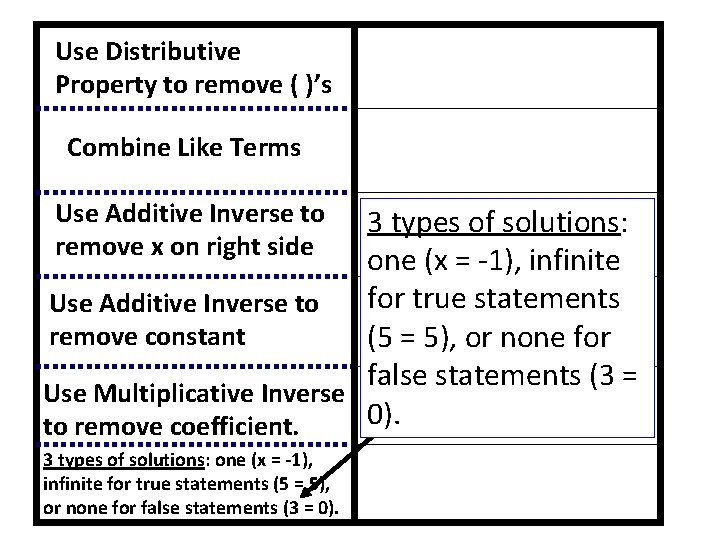Use Distributive Property to remove ( )’s Combine Like Terms Use Additive Inverse to remove x on right side 3 types of solutions: one (x = -1), infinite for true statements Use Additive Inverse to remove constant (5 = 5), or none for false statements (3 = Use Multiplicative Inverse 0). to remove coefficient. 3 types of solutions: one (x = -1), infinite for true statements (5 = 5), or none for false statements (3 = 0).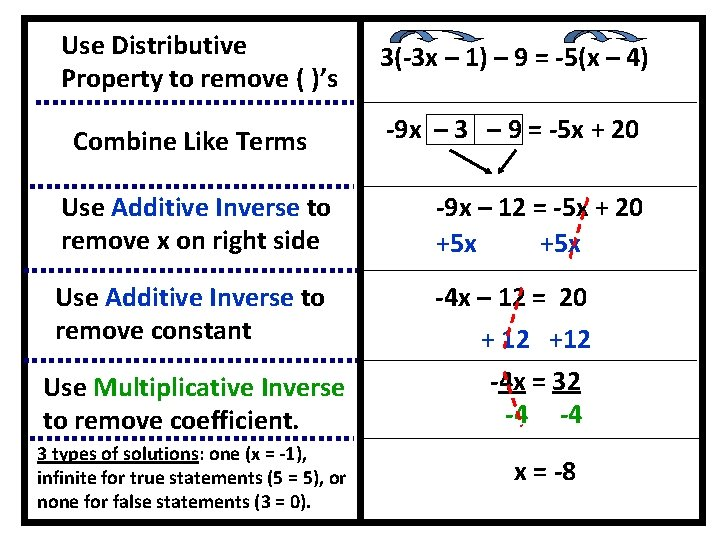Use Distributive Property to remove ( )’s Combine Like Terms 3(-3 x – 1) – 9 = -5(x – 4) -9 x – 3 – 9 = -5 x + 20 Use Additive Inverse to remove x on right side -9 x – 12 = -5 x + 20 +5 x Use Additive Inverse to remove constant -4 x – 12 = 20 + 12 +12 -4 x = 32 -4 -4 Use Multiplicative Inverse to remove coefficient. 3 types of solutions: one (x = -1), infinite for true statements (5 = 5), or none for false statements (3 = 0). x = -8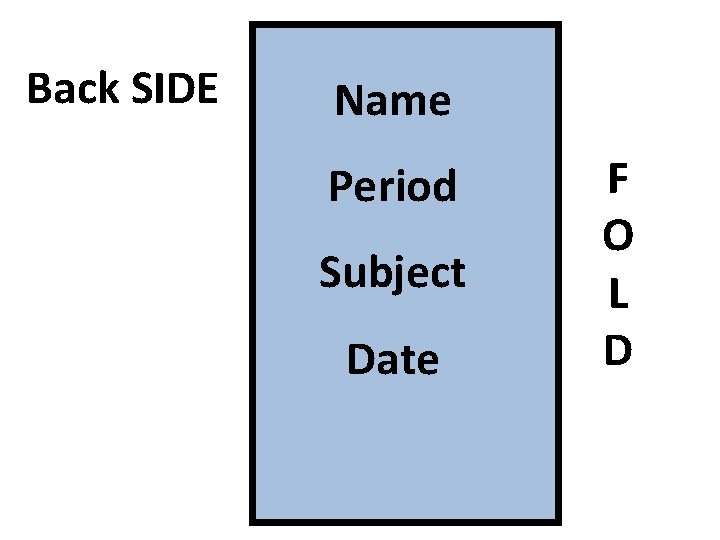Back SIDE Name Period Subject Date F O L D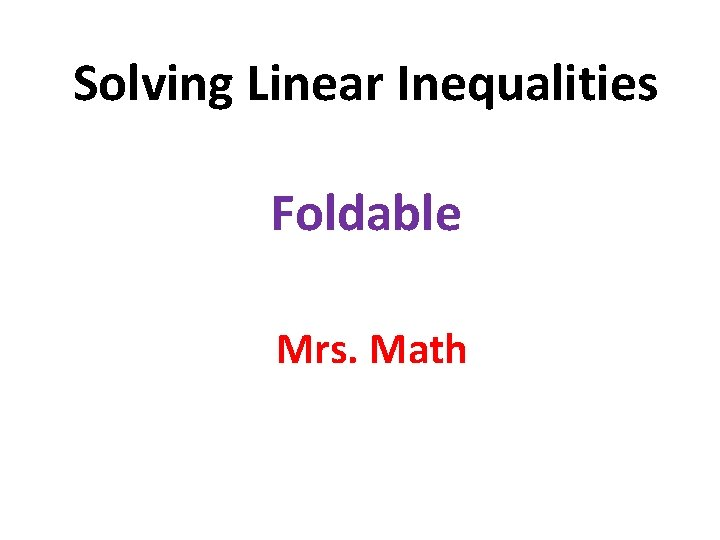Solving Linear Inequalities Foldable Mrs. MathSolve Inequalities Foldable Fold along the middle (hotdog style)Solve Inequalities Foldable Open fold and draw lines across the inside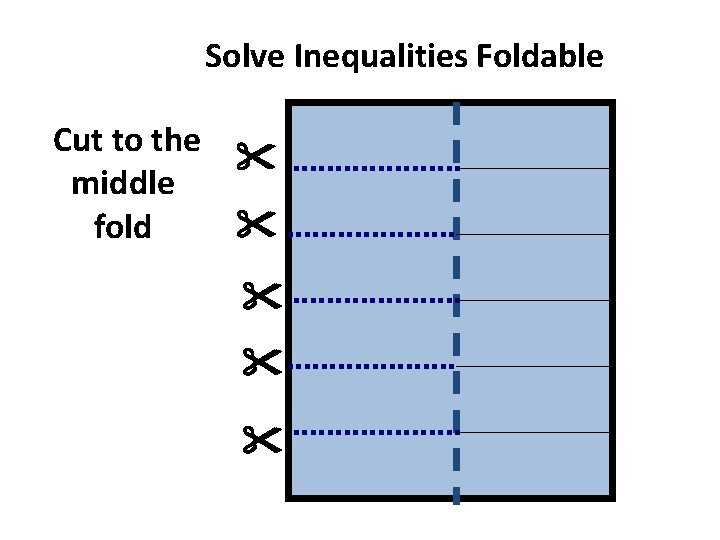Solve Inequalities Foldable Cut to the middle foldParenthesis ( )’s F O L D X’s on Same Side (or constants) X’s on Opposite Sides Constant Coefficient Inequality FRONT SIDE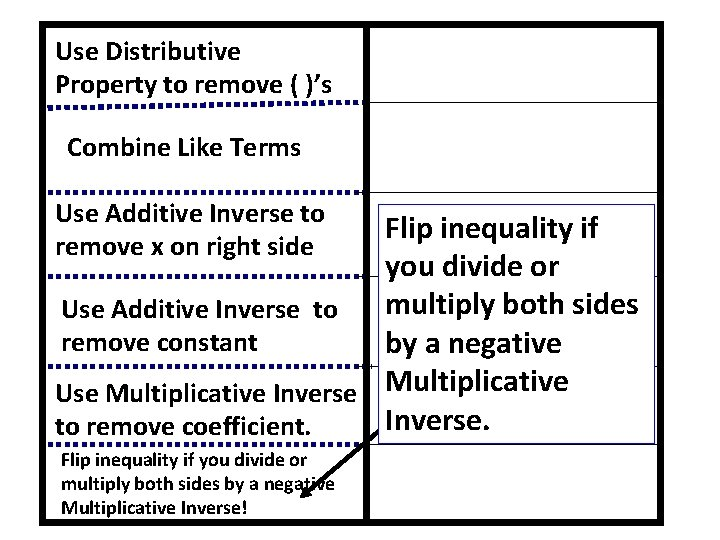Use Distributive Property to remove ( )’s Combine Like Terms Use Additive Inverse to remove x on right side Flip inequality if you divide or multiply both sides Use Additive Inverse to remove constant by a negative Use Multiplicative Inverse. to remove coefficient. Flip inequality if you divide or multiply both sides by a negative Multiplicative Inverse!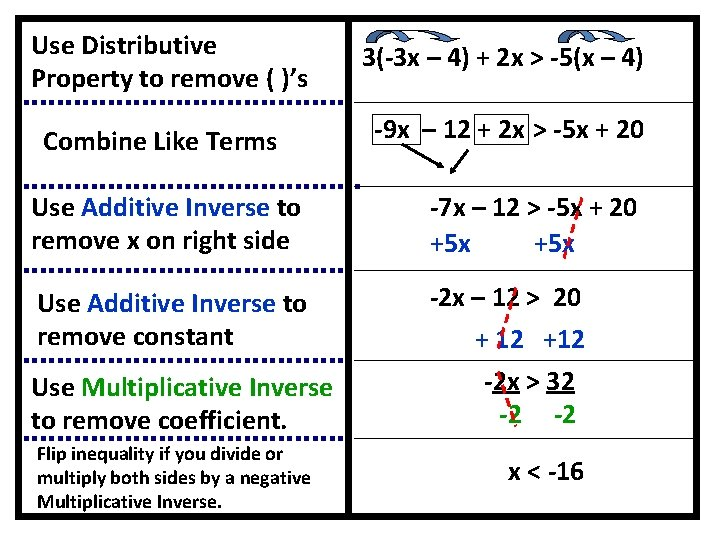Use Distributive Property to remove ( )’s Combine Like Terms 3(-3 x – 4) + 2 x > -5(x – 4) -9 x – 12 + 2 x > -5 x + 20 Use Additive Inverse to remove x on right side -7 x – 12 > -5 x + 20 +5 x Use Additive Inverse to remove constant -2 x – 12 > 20 + 12 +12 -2 x > 32 -2 -2 Use Multiplicative Inverse to remove coefficient. Flip inequality if you divide or multiply both sides by a negative Multiplicative Inverse. x < -16Back SIDE Name Period Subject Date F O L D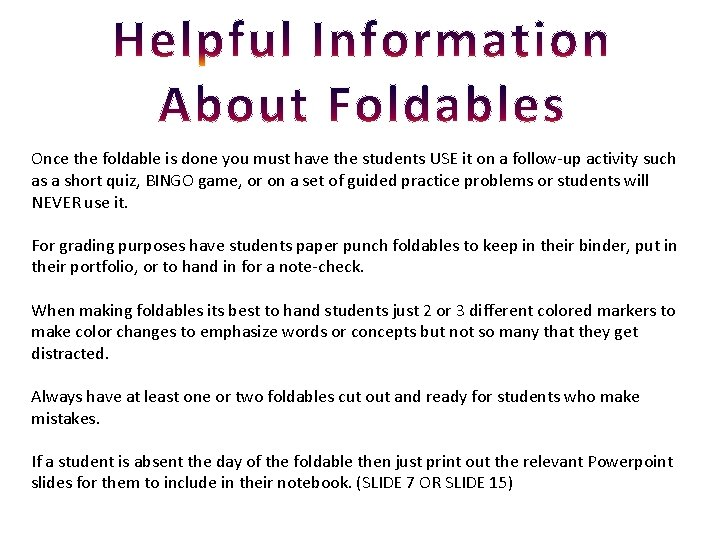Once the foldable is done you must have the students USE it on a follow-up activity such as a short quiz, BINGO game, or on a set of guided practice problems or students will NEVER use it. For grading purposes have students paper punch foldables to keep in their binder, put in their portfolio, or to hand in for a note-check. When making foldables its best to hand students just 2 or 3 different colored markers to make color changes to emphasize words or concepts but not so many that they get distracted. Always have at least one or two foldables cut out and ready for students who make mistakes. If a student is absent the day of the foldable then just print out the relevant Powerpoint slides for them to include in their notebook. (SLIDE 7 OR SLIDE 15)